## Introduction-

Today, let's learn about a famous and commonly asked Interview Problem, i.e., Breadth-First Search in a graph. It is a critical concept used to solve various significant problems of graphs.

It is very similar to the Level Order Traversal of a Tree.

In a tree, we cannot revisit the parent node and cannot have a self-loop. But there is no such restriction in the Graph.

Example:

Let's consider this Graph and try to perform a Breadth-First Search(BFS) on the graph.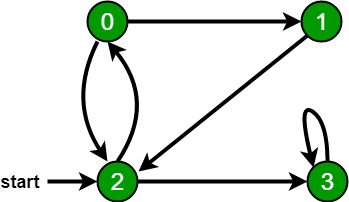For calculating the Breadth-First Search, we are always given the source vertex i,e. The start vertex. Here, 2 is our source code.

Like level order traversal, we will discover all the nodes at the same level in Breadth-First Search.

So, first, we visit 2 (our source node) and then all its neighboring nodes, i.e., 0 and 3.

Now, we come to the next level and discover all the neighboring nodes of  0, i.e., 2 and 1.

## Importance of Visited Array

It is vital in graphs as a node can visit any other node. As a result, we would keep visiting the node at the previous level and soon be stuck in an infinite loop.

Like in the above example, if we don't keep a visited array, 2 would add 0 as its neighboring node. 0 would add 2, and again 2 would add 0 and so on.

However, keeping in the visited array helps prevent this. Once a node's neighbors are visited, it is marked as visited and no longer added to our queue.

## Approach

We would maintain a queue data structure and the visited array to store all the Graph nodes.

Principle of Breadth-First Search: remove - mark visited - add node - mark visited (r m* am*)

So, After adding the source node in the queue, mark it as visited.

Then in the while loop (till the queue is not empty) -

1. Remove the front node from the queue.
2. Check if it is visited - if yes: continue as work for the current node is already done, else mark it visited.
3. Run a loop and add all the neighbors of the node in the queue if that neighbor is not visited.

Continue this same process until the queue is empty.

Let's do a dry run on an example:

Let the start vertex be 0. Initially, the visited array and the queue are empty.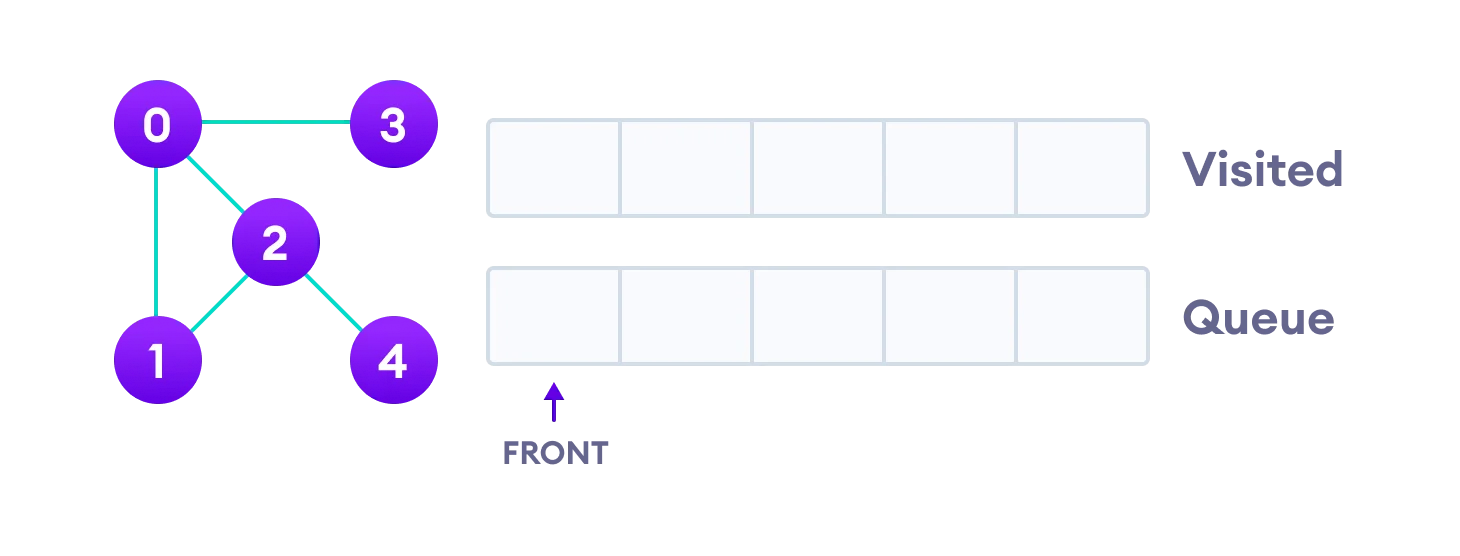Step1: Check if the start Vertex is null. SInce, start vertex is not null, it is added to the queue. So, the queue 

Now, we work while the queue is not empty.

Step2: Front element i.e 0 is removed from the queue.

Step3: Since 0 is not visited, it is marked visited.

Step4: 0’s unvisited adjacent vertex i.e. 1, 2 and 3 are added in the queue.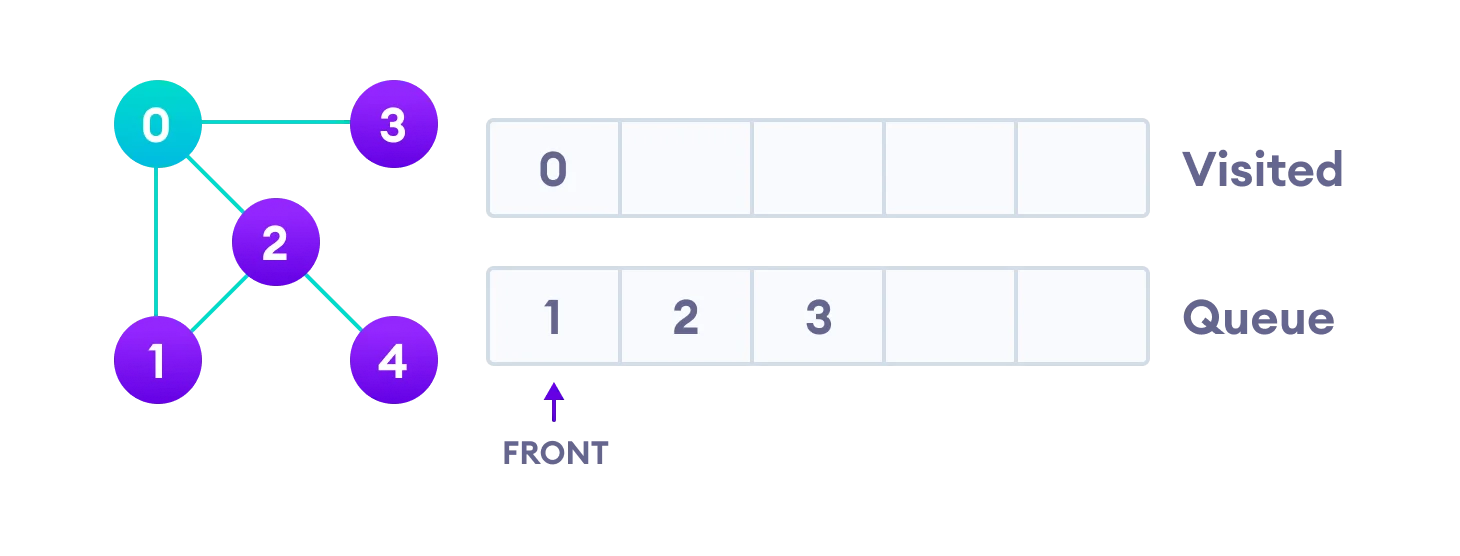Step5: Front element i.e 1 is removed from the queue.

Step6: Since 1 is not visited, it is marked visited.

Step7: 1’s unvisited adjacent vertices i.e. 2 is added in the queue.=> Queue [2,3,2]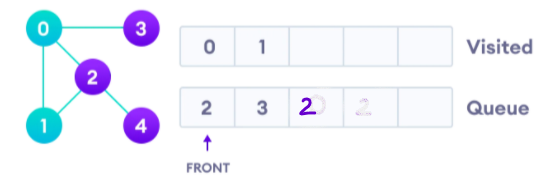Step8: Front element i.e 2 is removed from the queue.

Step9: Since 2 is not visited, it is marked visited.

Step 10: 2’s unvisited adjacent vertices i.e. 4 are added in the queue.=> Queue [3,2,4]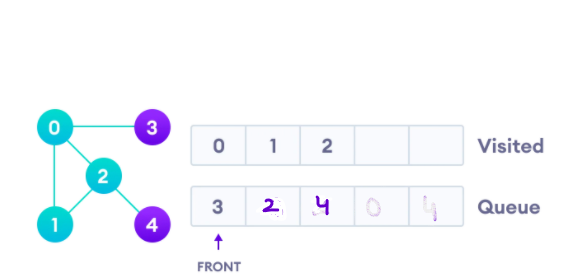Step11: Front element i.e 3 is removed from the queue.

Step12: Since 3 is not visited, it is marked visited.

Step13: 3 has no unvisited adjacent vertex. => Queue [2,4]

Step14: Front element i.e 2 is removed from the queue.

Step15: Since 2 is visited, the loop is continued.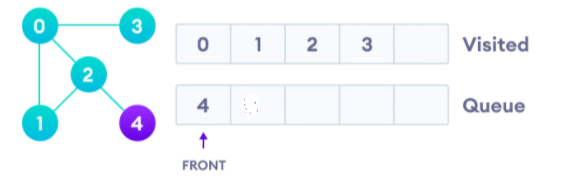Step16: Front element i.e 4 is removed from the queue.

Step17: Since 4 is not visited, it is marked visited.

Step18: 4 has no unvisited adjacent vertex.=> Queue []

Now, the queue is empty and the loop ends.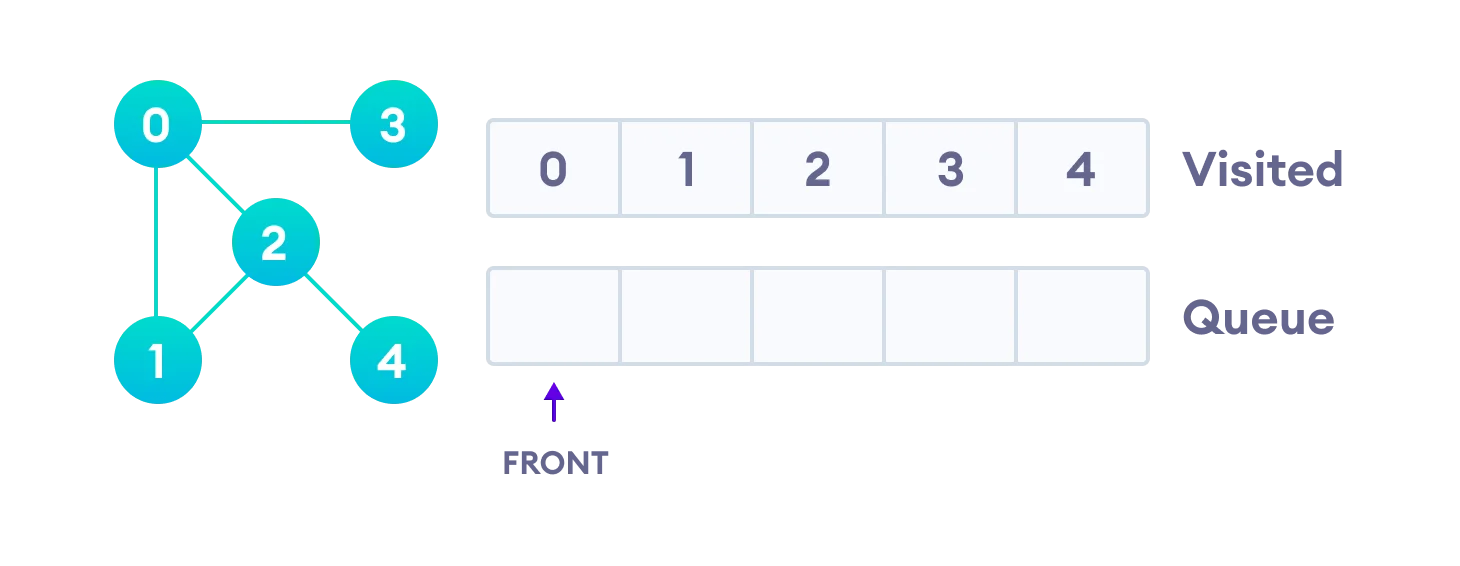Implementation-

Let’s have a look at its implementation in Java -

Output:

## Time and Space Complexity-

Time Complexity:  O(E+V) as we are traversing all the nodes of the Graph for finding the Breadth-First Search of a graph once.

Space Complexity: O(V) as extra space for storing nodes in a queue as well as the visited array of O(V) size is being used.

Where V is the number of vertices in the graph.

E is the number of edges in the graph.

1. What is a Graph?

Ans. A Graph is a non-linear data structure consisting of nodes and edges. The nodes are sometimes also referred to as vertices and the edges are lines or arcs that connect any two nodes in the graph.

2.  What is the Breadth-First Search?

Ans: Breadth-First Search is an algorithm for searching a tree data structure for a node that satisfies a given property. It starts at the tree root and explores all nodes at the present depth prior to moving on to the nodes at the next depth level.

3.  What is the best case time complexity for finding the Breadth-First Search in a Graph?

Ans. The best-case time complexity for finding Breadth-First Search in a graph is O(1), i.e. when only a single or no vertix is present in the Graph.

## Key Takeaways-

In this blog, we learned about the Breadth First Search of a graph.

• Maintain a queue data structure and the visited array to store all the Graph nodes.
• Principle of Breadth-First Search: remove - mark visited - add node - mark visited (r m* am*)
• Maintaining a visited array is important to prevent the possible infinite loop.
• The minimum time complexity required is O(V+E) where v = number of vertices and e = number of edges in a Graph as we need to traverse all the nodes of the Graph once.

Check out more blogs on various different traversals and questions of Graphs like Depth First Traversal, Level Order Traversal to read more about these topics in detail. And if you liked this blog, share it with your friends!